A collection of physics problems with subtly flawed solutions. Try to find the flaw!

Rules: “we know” means definitely true. Everything else is questionable.

Credit sources (eg, Morin, Griffiths) if not common knowledge.

Falling Blocks [Dynamics, Gravity] (Easy)

Consider gluing a heavy brick besides a light brick.

When the combination is dropped (ignoring air resistance), we know the chunk will not rotate while falling.

But, considering bricks individually, the force of gravity on the heavy brick (Mg) is greater than the force of gravity on the light brick (mg):This causes a torque, which in turn causes the chunk to spin -- why doesn’t it?

Extra: Explain what happens with air-resistance (bricks identical in shape), and why.

Inseparable Horseshoes [Gravitation] (Easy)

Take a horseshoe. We know that the center of mass will be somewhere in the space between the two arms.

Take two horseshoes and facing eachother so that the ends are touching ⊂⊃. Now rotate one of them 90º around the axis parallel to the bar and through the center of mass – ⊃ and move them together until the center of masses perfectly overlap.

We know that Fgrav = G(Mm)/r^2. Since the distance between the center of mass is now 0 this force should be infinite and the horseshoes should be permanently stuck. Why aren’t all horseshoes permanently gravitationally locked?

Two Liquids in a tube [Fluids] (Easy)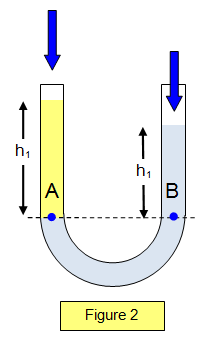Note: another variant of Falling Blocks.

Consider two liquids with different densities as shown. In general, the final heights from the base of the two liquids in equilibrium need not be the same. However, one can make the following argument:

Suppose the heights aren’t equal as shown where liquid A is higher. Consider the the liquid B and the liquid A up to the height of B as one object (call it C) and call the rest of A, D. We can see that D will exert a force on object C causing it to flow to the right. This will push the liquids to the same height. What went wrong here?

Evel Knievel [Dynamics] (Medium)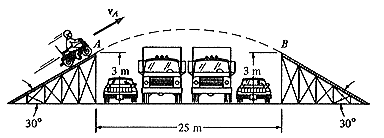Consider the motorcycle jump above. The original problem asked for the speed v_A for which the landing velocity would be tangent to the ramp (as drawn), making for a smooth landing. But we should also require that orientation of the cycle upon landing be tangent to the ramp (so the motorcycle lands on both wheels).

We notice that in footage of car/motorcycle jumps, the vehicle seems to rotate naturally. But what causes this? There are no significant torques on the vehicle in midair…

If you think you know, work out the details. Provided the launch/landing ramps are symmetric, under what conditions will “natural” jumps be possible? (This may depend on characteristics of the vehicle. No air-resistance for simplicity).

Note: It is possible for a vehicle to change its angular position in mid-air, for example by revving its wheels. Let us ignore this possibility as the norm, since the rotational motion seems to occur “naturally” without such actions.

Spinning Ice-Skater: A closer look [Dynamics] (Medium)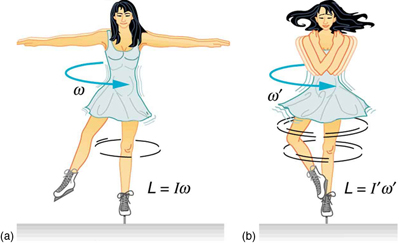We know that when a spinning ice-skater pulls in her hands, she spins faster. This is justified by conservation of angular momentum. But let us look more closely:

Consider the dancer in two parts: her core and her arms. For simplicity, let the dancer pull her arms straight inwards towards her. Since her core’s angular velocity (and hence, angular momentum) increases, we know there must be a torque on her core. In particular, this torque must be caused by her arms. But how can her arms exert a torque on her core, if she pulls them straight inwards? (Because, if the force on her arms by her core is always radial, then her arms can exert no torque on her core!)

Said more simply: Pulling my arms in when I am stationary does not cause me to spin. So why does pulling my arms in when I’m already spinning cause me to spin faster?

Remark: In the rotating frame of the dancer, you’ll see the Coriolis force on her arms causing an apparent torque.

Stacking Blocks [Statics] (Medium)

How far away from an edge can you stack blocks given an infinite number of blocks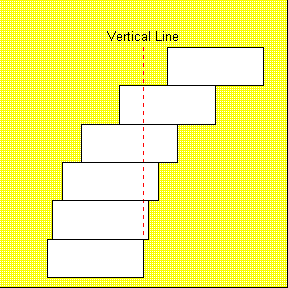The length of one block is d

let us now define the length of our stack as L(n) where n is the number of blocks

trivially we will define L(1) = d

creating our stack we will place the lower block such that the upper center of mass is exactly on the edge thus pushing the bounds of stability

the center of mass for our single block is half way down (at distance d/2) so we place our lower block so that d/2 of the upper block overlaps.

Our new stack has two blocks and a total length of 3/2 d. The center of mass is halfway along this length so when we add another lower block we get the recurrence relationship

L(n+1) = L(n)/2 + d

because the center of mass is at L(n)/2 and we are adding d to that position

this gives us values of 1, 3/2, 7/4 etc and matches the exponential

L = d(2-½^(n-1)) which approaches 2*d as n->∞ meaning in the limit our stack can be twice as long as a single block. But the picture gives a balanced arrangement with >2d extension -- what went wrong?

Master solution:

(@nolan: must be a simpler way to derive recurrence)

Consider a right-leaning stack. Normalize the block-length to 2 for simplicity.

Let P(n) = (CM position of the n’th block, relative to the right-edge of the 1st -- topmost -- block).

P(1) = 1

P(2) = 2

P(3) = 5/2

The bottom (n+1)th block’s right-edge must be at the CM of the upper (n) blocks. So:

P(n+1) = 1/n * \sum_{i=1}^n P(i) + 1

We can confirm that:

P(n+1) - P(n) = 1/n

Or derive it by noticing: nP(n+1) - P(n) = (n-1)P(n) + 1

So P(n) ~ H_n

(harmonic sum)

Extra:

If you solved the above properly, we know that it is in fact possible to achieve an infinite-extent of stacked blocks. Consider standing at the bottom of such a stack, looking up (WLOG, let the blocks be stacked leaning right). At some point, the CM of a stacked block must cross the right edge of the bottommost block (since the stack is infinite in extent). Above this point, we can only have a finite number of blocks (since if we had an infinite #, we would have infinite mass to the right of the base support, causing the CM of the entire structure to be outside the base). But a finite number of blocks have a finite extent -- so how can our stack support an infinite extent?

Rolling to a Stop [Dynamics] (Easy -- conceptual)

Why do rolling balls stop?

If you say “friction”, be careful -- friction where and why?

The ball rolls without sliding, so kinetic friction from sliding is ruled out…

(Consider the dominant factor in several cases: deformable ball, deformable surface, bumpy surface, bumpy ball).

Running and Falling [Dynamics] (Easy)

When running, friction from the ground on your feet provides forward force (after all, you “push off” from the ground).

But this causes a torque about your CM -- why do you not fall over when running?

Circular Fiction [Dynamics] (Hard -- at least, requires proper exposure)

Put a tennis-ball inside a frictionless tube, and spin the tube about one end (not along its axis).

We know that the tennis-ball will fly out the other end.

But what forces accelerate the ball? The normal force is always perpendicular to the radius.

Be careful here… no fictitious forces.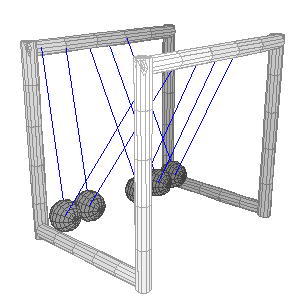We know that in Newton’s cradle, dropping two balls together from one side results in two balls symmetrically rising on the other side. This is usually explained by appealing to conservation of momentum/energy. But in fact, the solutions are degenerate:

If two balls of unit mass are dropped together such that they collide with unit velocity, it is possible for three balls to rise, with the following velocities:

v_1 = 4/3

v_2 = 1/3

v_3 = 1/3

Confirm that this solution also satisfies momentum/energy conservation. Why does this not occur?

Action at a Distance [Misc] (Trivial)

Imagine a communication system involving a 10-km long rigid metal rod, that is pulled back/forth to communicate 1/0s between operators at both ends. This instantly communicates information over the 10-km span -- why is this faster-than-light communication scheme not in use?

Holey Sheet [Thermal] (Easy/Medium)

Consider the familiar puzzle of what happens to a flat sheet of metal with a hole in it, when the sheet is heated. We know that the hole (along with the rest of the sheet) will expand in size.

But let us take a closer look:

Consider an infinitesimal area element of the sheet.

When heated, this element will attempt to expand -- and will exert forces on its boundaries in the process.This explains why the entire sheet expands: The metal on the boundary of the sheet experiences outward force from the interior metal expansion, which is not balanced by outside forces (since, there is no metal beyond the boundary to push back).

By similar reasoning, the metal on the boundary of the hole has nothing to balance the inward forces form the exterior metal expansion, so the hole should contract.What breaks the symmetry between the interior and exterior? Why does the hole expand?

Expanding Solids [Thermal] (Medium)

We generally assume that atoms in a solid are masses on a spring moving in SHM, and all its properties can be determined using this model. In that case, if we heat a solid the atoms should still execute the same SHM, but with increased amplitude - that is, its equilibrium point should be unaltered. Why then do atoms move away from each other on heating, resulting in expansion of the solid ?

There are two runners - one slow (A) and one fast (B). The faster runner feels pity for the slower person and gives him/her a head start during a race. Say A travels a distance d1 before B started. B will take some time to cover the distance d1 to catch up with A. In the meantime, A would have travelled a distance d2 further. So, B has to catch up with A again, and this process repeats. This means B will always have to be trying to catch up with A. So, if A gets a head start, will he will always stay ahead and inevitably win ?

Reversing a straw [Fluids] (Hard)

A vertical straw is filled with water with the top end held by a finger. If we let our finger go, the stream of water accelerates downwards from gravity, reducing its cross-section outside the straw to preserve continuity.
Now suppose we are free-falling along with the water. We should see the straw accelerating upward at a rate g, and the stationary water is reducing its cross section by itself! What’s going on?

Spinning U-tube[Fluids](Easy)A U-tube is spun along the off-centered axis shown. Since the longer side has more centrifugal force. The height on the longer side will be greater.
However, using Bernoulli’s equation,
ρv_1^2/2+ρgh_1 = ρv_2^2/2+ρgh_2, and v=ωr we would obtain that the height of the shorter side would be greater (and in fact, the height difference calculated in the two cases will be exactly the same.) Explain which method is correct and why the other method is wrong?

Osmotic Pressure [Fluids] (Easy/Medium)

Consider a U-tube, with equal volumes pure water (on the left) and salinated water (on the right), separated by a semi-permeable membrane. We know that from this initial condition, water will transfer from the left to the right halves of the tube, along the osmotic pressure gradient.

This will result in unqeual levels of water in both halves, in equilibrium.

Classical physical systems tend to approach states of lowest potential energy.

But consider the gravitational potential energy of the initial and final configurations -- the final equilibrium is in fact at higher potential energy. Further, the initial configuration is the lowest possible potential energy, yet it is not a stable equilibrium. Where does the energy approach break down?

Electromagicians [E&M] (Hard)
Carefully justify the use of “voltage” in any circuit that involves magnetic elements (for example, inductors). We know that voltage is only well-defined when the curl of the E field is zero (<==> no changing magnetic fields). And yet electrical engineers use V = L di/dt all the time, and seem to get meaningful results. What is the basis for this usage, and does it have any limitations? (any cases when it won’t work, or give nonsensical results).

Consider a planet, orbiting the (very massive) sun. We know that applying a radial impulse (that is, an impulse directed toward the sun) to the planet will not affect its total angular momentum, because it exerts no torque. But, this radial impulse will move the planet closer to the sun, while not affecting its tangential velocity. L = r x p = r x p_tang, and this impulse will decrease ‘r’ while keeping the same tangential velocity/momentum --- why doesn’t L change?

Carefully follow the derivation for Hamilton’s principle of stationary action, with constraint forces. In particular, carefully define what it means for a force to be a force-of-constraint. (The common answer is that “constraint forces do no work.” But this is not true -- consider a pendulum in an elevator, force to accelerate upwards. In this case, the constraint force of the pendulum-string clearly does work on the bob.)Trajectory Instability [Dynamics] (Easy/Medium)

Consider throwing a ball up in the air two ways: first straight up, then slightly to the side.

Let us examine the full trajectory (/orbit) of this ball, if it never hit the ground (that is, if the Earth was a point mass as usual).

When we throw it straight up, we expect it to oscillate about the center of the earth (RIGHT)

And when we throw it slightly to the side, we expect a slight ellipse (LEFT)

We know that in (non-degenerate) Kepler orbits, the center of mass (~ center of the earth) lies at a focus of all orbits. But this is not the case for the orbit to the left. What does the correct orbit look like? Discuss its dissimilarity to the case when we throw the ball straight up (zero angular momentum)... in particular, it does not make it very far toward the other side of the earth.

Millstone Gyro [adapted from K&K Problem 7.4] (Medium)

Consider the below milling wheel, with a heavy grindstone. An analysis of angular momentum (dL/dt) about the intersection of both axles shows that the normal force on the millstone is greater than its weight. (This is essentially a gyroscopic effect).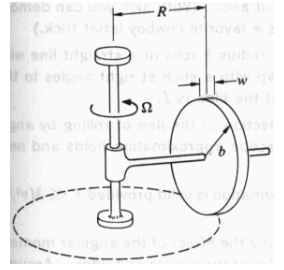But, consider force-balance on the wheel -- how is the millstone in equilibrium if the normal force (from the ground) upwards exceeds gravity downwards?

Hund’s rule [Atomic Physics/QM] (Easy/Hard)

We know that the orbitals (except s) are not totally degenerate in energy and can be arranged into separate energy levels. Also from Pauli’s exclusion principle, we know that we cannot have two electrons of the same spin - i.e if two of them are in an orbital, they must have opposite spins. So, we would expect the electrons to fill up the lower energy orbital, with electrons of both spins present to minimize total energy. However, From Hund’s rule of maximum multiplicity, it is known that when filling up orbitals of a subshell, the system prefers aligning spins in the same direction (In most sane cases). Following Hund’s rule, we should be having a higher energy. Why then do electrons still fill up in the way predicted by Hund’s rule ?

Easy - What is a phenomenon left out, which when included makes Hund’s rule sensible ?

Hard - Why does the phenomenon explain Hund’s rule (Will require a good understanding of QM) ?

(NOTE - I think this question is not phrased very well; corrections/suggestions are welcome)

Rotating plate [misc/fluids] (Medium)

Suppose you have a square plate with its left half painted black and the right half white. Suspend this in a transparent container which is evacuated (using a laboratory vacuum pump). Suppose we shine light (produced by some black body) uniformly on the plate, we should expect it to rotate counter-clockwise due to radiation pressure: the light is absorbed by the black side, but reflected by the white side. It therefore imparts greater momentum to the white side, which should make it rotate counter-clockwise.

Experiments however show that this system (in the configuration described above) rotates clockwise instead. Why does it behave contrary to our expectations ?

Note - If you think you have the answer, think again.

Constants of Motion [Advanced Mechanics] (Medium)

Given a Lagrangian, why is it not valid to plug in known constants of motion into the Lagrangian (before differentiating)? Ie, why does this result in different EOMs?

Example: In central force motion:

L = ½\mu \dot r^2 + ½ \mu r^2 \dot \phi^2 - U(r)

But we know the angular momentum l_z = (\mu r^2 \dot\phi) is constant, so why can’t we use this to eliminate \dot\phi in the Lagrangian? (If we do this, the EOM we get has a sign flip)

TODO: simpler example?

The Lagrangian should describe the action of a system along all possible trajectories. By finding stationary-action trajectories, we recover the true path of a system. However, the constants of motion (eg, angular momentum) are only constant along the actual trajectory -- so if we plug them in, the resulting Lagrangian will only be valid along the system’s true path. It is not valid to vary this Lagrangian along other paths, so we do not recover the proper EOMs.

Mass on Heavy Spring [Dynamics] (Hard)

Consider a SHO, mass (M) on a spring (k) -- but now do not neglect the mass of the spring itself (m). Conventional wisdom says that the correction due to the spring’s “effective mass” is simply to add +m/3 to the inertia of the system. The usual derivation is straightforward, for example:

http://en.wikipedia.org/wiki/Effective_mass_(spring%E2%80%93mass_system)

The crucial assumption made above (and in most animations of such systems) is that the spring remains “evenly stretched” throughout its motion. But can this be true?

To simplify, just consider a horizontal system (no gravity), and a spring with some rest length L.

If the spring indeed remains equally stretched (<==> uniform tension), then an infinitesimal spring element will feel no net force (tensions to left and to right will perfectly cancel). Thus it will not accelerate. But we know the spring oscillates, so the tension cannot remain uniform.

So what actually happens? Describe the behavior of the spring. (Make any approximations or simplifying assumptions, but be sure they are physically consistent…. unlike the “uniform tension” assumption).

Answer: See this for a fully rigorous (but perhaps not very illuminating) treatement: http://people.westminstercollege.edu/faculty/ccline/courses/phys305/shm-effective_mass_2.pdf

TODO: illuminate

+ light between mirrors

+ E&M: magnetic forces doing “work”

+ Magnetic forces violates Newton’s 3rd law

+ select perpetual motion machines

+ mirrors flipping left to right not up to down

+ peak frequency * peak wavelength from black body radiation != c

+ reference to the classics? (“Feynman sprinkler”)

+ “Understanding the Chain Fountain”: http://arxiv.org/pdf/1310.4056v2.pdf